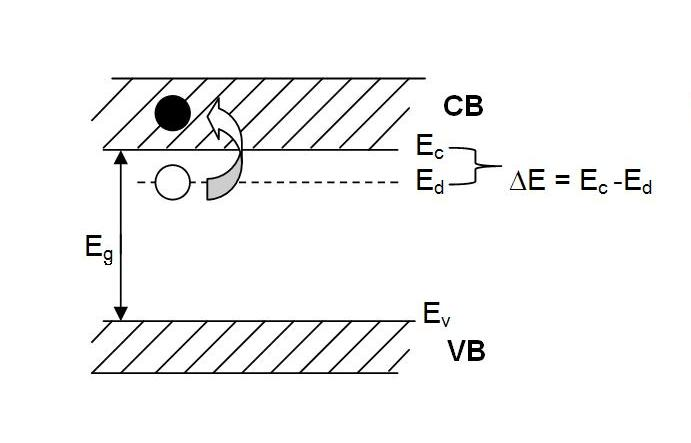$\newcommand{\dif}{\mathop{}\!\mathrm{d}} \newcommand{\p}{\partial}$

1. 间隙式杂质：原子半径小
2. 替位式杂质：原子半径大

# 施主杂质与施主能级1. 比成键电子自由，$E_D » E_V$
2. 与导带电子有差别（受到 $P^+$ 库仑力作用），$E_D=E_C-E_{库伦}$1. 杂质比较少，它的能级是孤立的，未能形成能带，称为孤立能级，用短线表示
2. $\Delta E_D « E_g$
1. 当 T=0K，束缚态
2. T≠0K，能带角度：电子从 $E_D$ 跃迁到 $E_C$；空间角度：电子脱离 $P^+$ 离子库仑力束缚，成为自由电子。此时的施主杂质处于 离化态

# 受主能级# 杂质浅能级电离能的简单计算

1. $\varepsilon_0\rightarrow \varepsilon_0\varepsilon_r$，对于硅：$\varepsilon_r(Si)=12$，对于锗：$\varepsilon_r(Ge)=16$
2. $m_0\rightarrow m^*$，作各向同性处理后：$\frac{1}{m^*}=\frac{1}{3}(\frac{1}{m_l}+\frac{2}{m_l})$

$E_n=\frac{(m^*/m_0)}{\varepsilon_r^2}\frac{13.6}{n^2}$

# 杂质的补偿作用

1. 空间角度：施主周围多余的价电子提供给受主，补偿受主的价键，使价键饱和，达到稳定状态
2. 能带角度：定义
1. 有效施主浓度（有效参杂浓度）：$N_{D(eff)}=N_D-N_A$
2. 杂质补偿度：$\gamma=1-\lvert \frac{N_D-N_A}{N_D+N_A} \rvert$，当刚好$N_D=N_A$时，$\gamma=1$，此时载流子浓度很低，几乎和本征半导体一样。

# 深能级杂质

1. 杂质能级离带边（导带底、价带顶）较远，$\Delta E_D, \Delta E_A \approx E_g$
2. 可多次电离，形成多重能级，甚至成为两性杂质。

1. $\Delta E_D, \Delta E_A$ 较大，对载流子浓度的影响较小
2. 对载流子的复合作用较大（复合中心），降低非平衡载流子的寿命，影响器件性能

# Ⅲ-Ⅴ族化合物种的杂质能级

1. Ⅱ族：取代Ga，成为浅受主
2. Ⅲ族：取代Ga，成为等电子杂质
3. Ⅳ族：既可取代 Ga，也可取代 As，成为两性杂质。一开始趋向施主，逐渐小部分成为受主，最后自己和自己补偿。
4. Ⅴ族：取代As，成为等电子杂质
5. Ⅵ族：取代As，成为浅施主